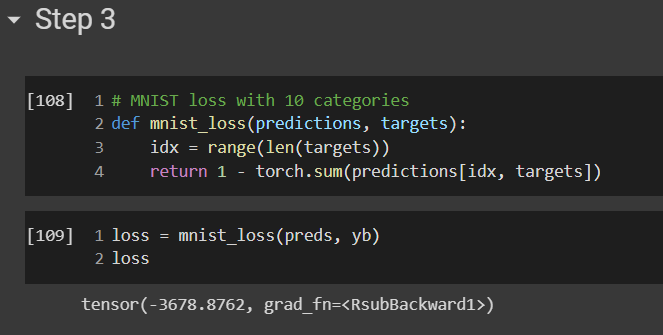# Lesson 4 Further Research 2, accuracy increases only when gradient is added

The accuracy actually decreases when I subtract the gradient as in the lesson, but it works perfectly when I add the gradient.

Hi Fazil,

There is something wrong with the loss function you are using.

If you use the one from the reference notebook, you will have a different result. As shown on this screenshot. The trick is that, the predictions is the loss when target==0 but when target==1, it is actually 1-prediction.Hope it helps !

Charles

1 Like

Hi Charles,
what you’ve posted is the correct loss function for MNIST with two categories, it doesn’t work for ten categories, but thanks to your post I found out what the problem is.I should’ve taken (1 - the sum) or the negative of it, so thank you